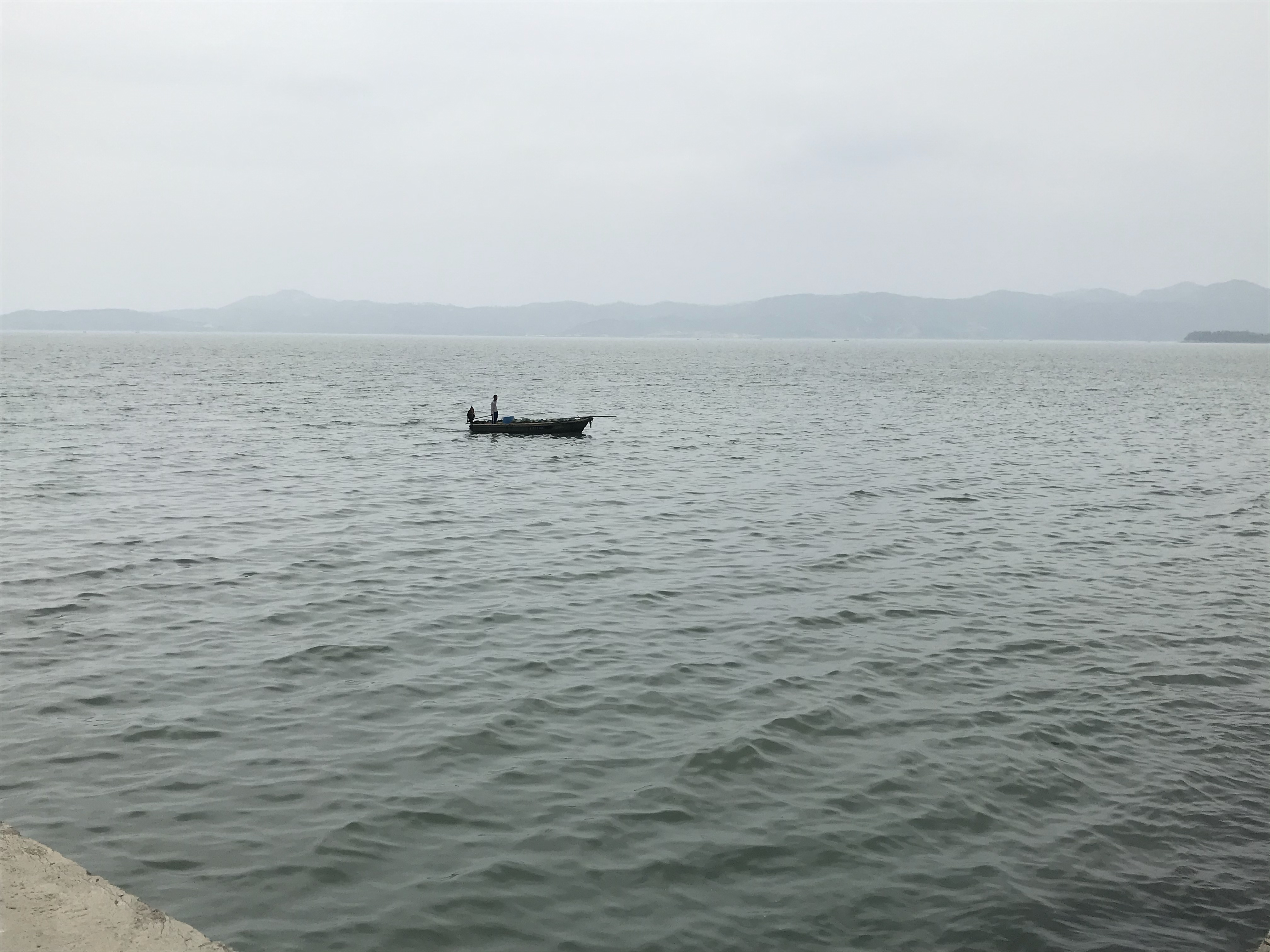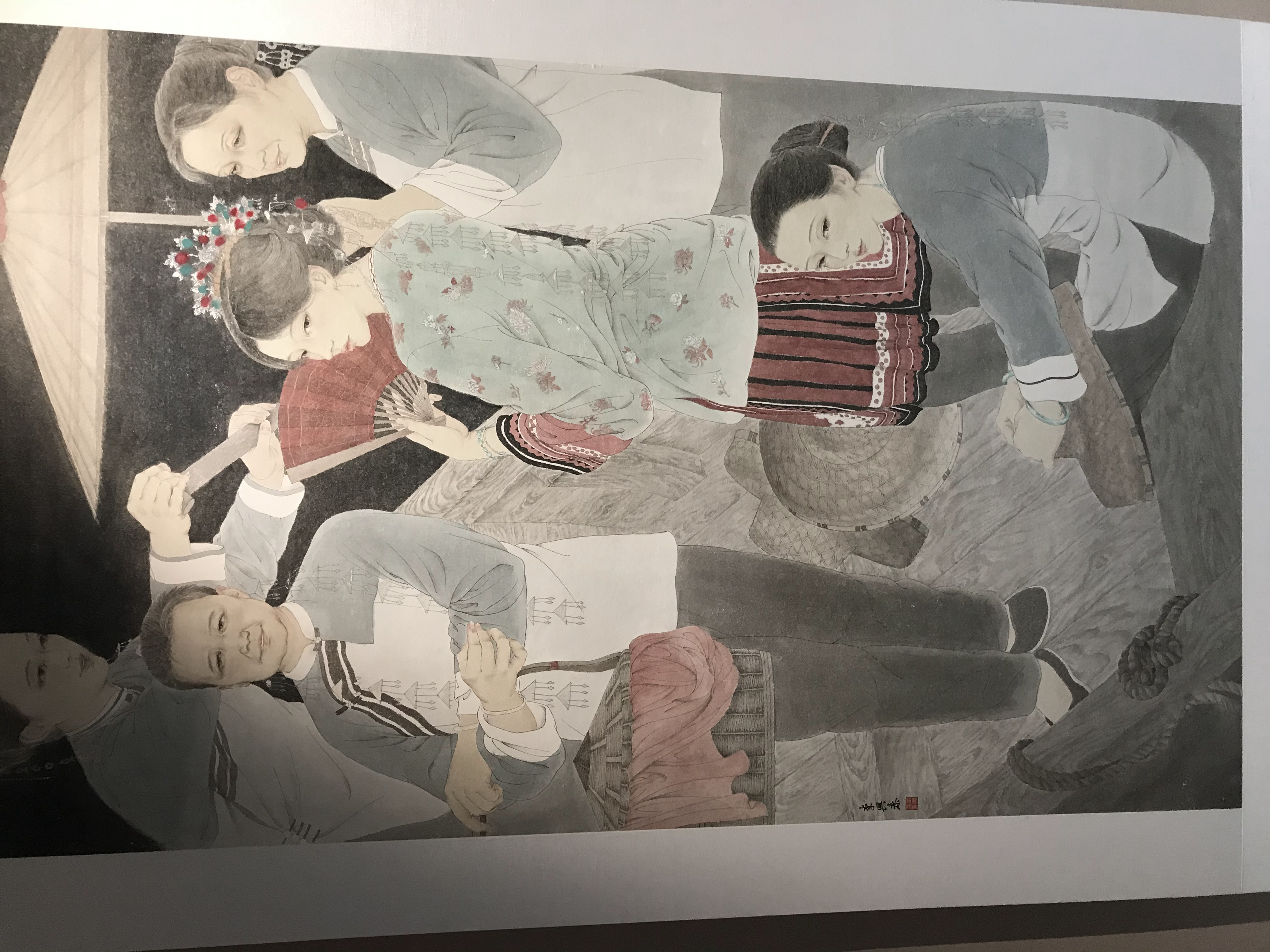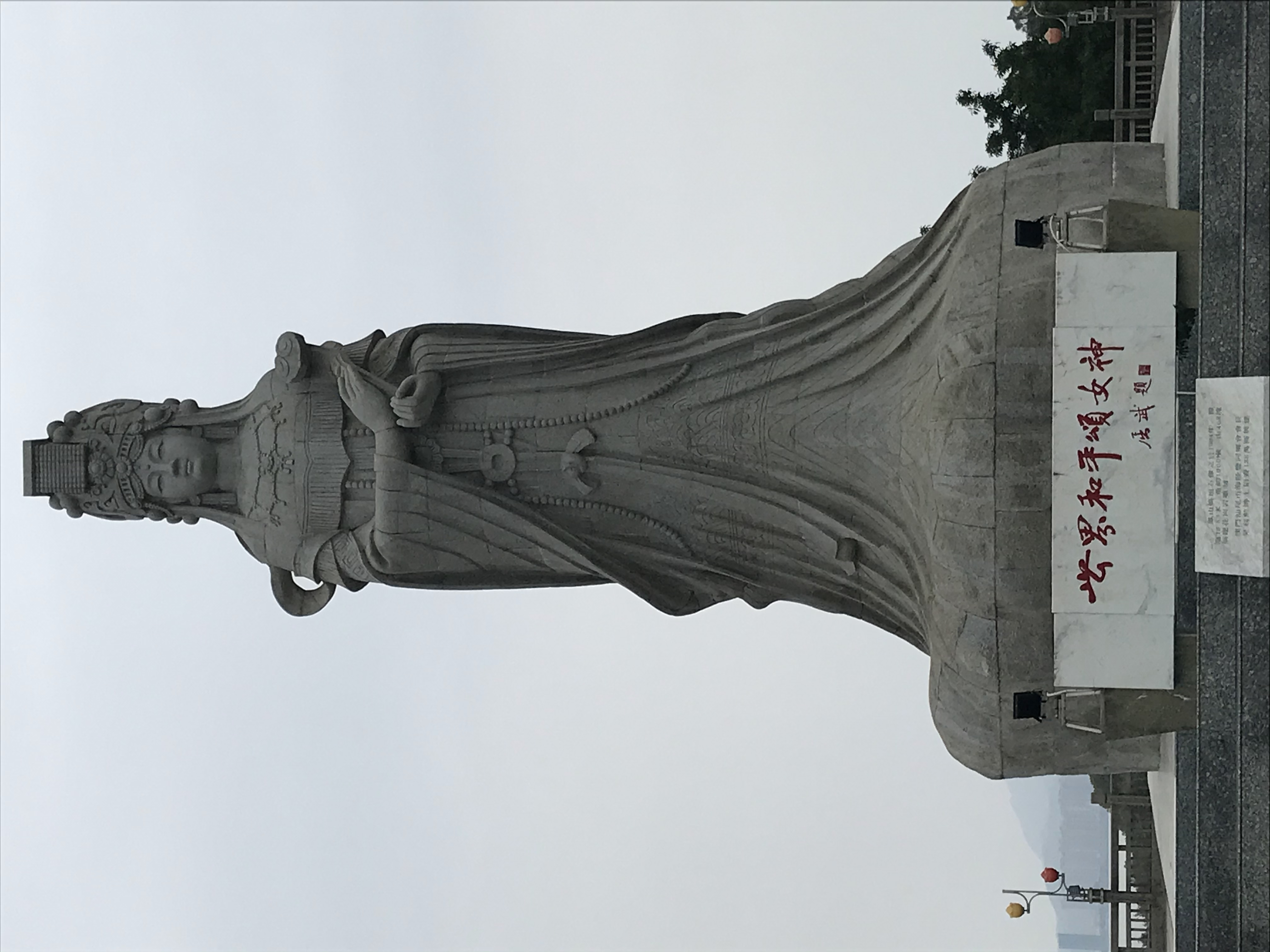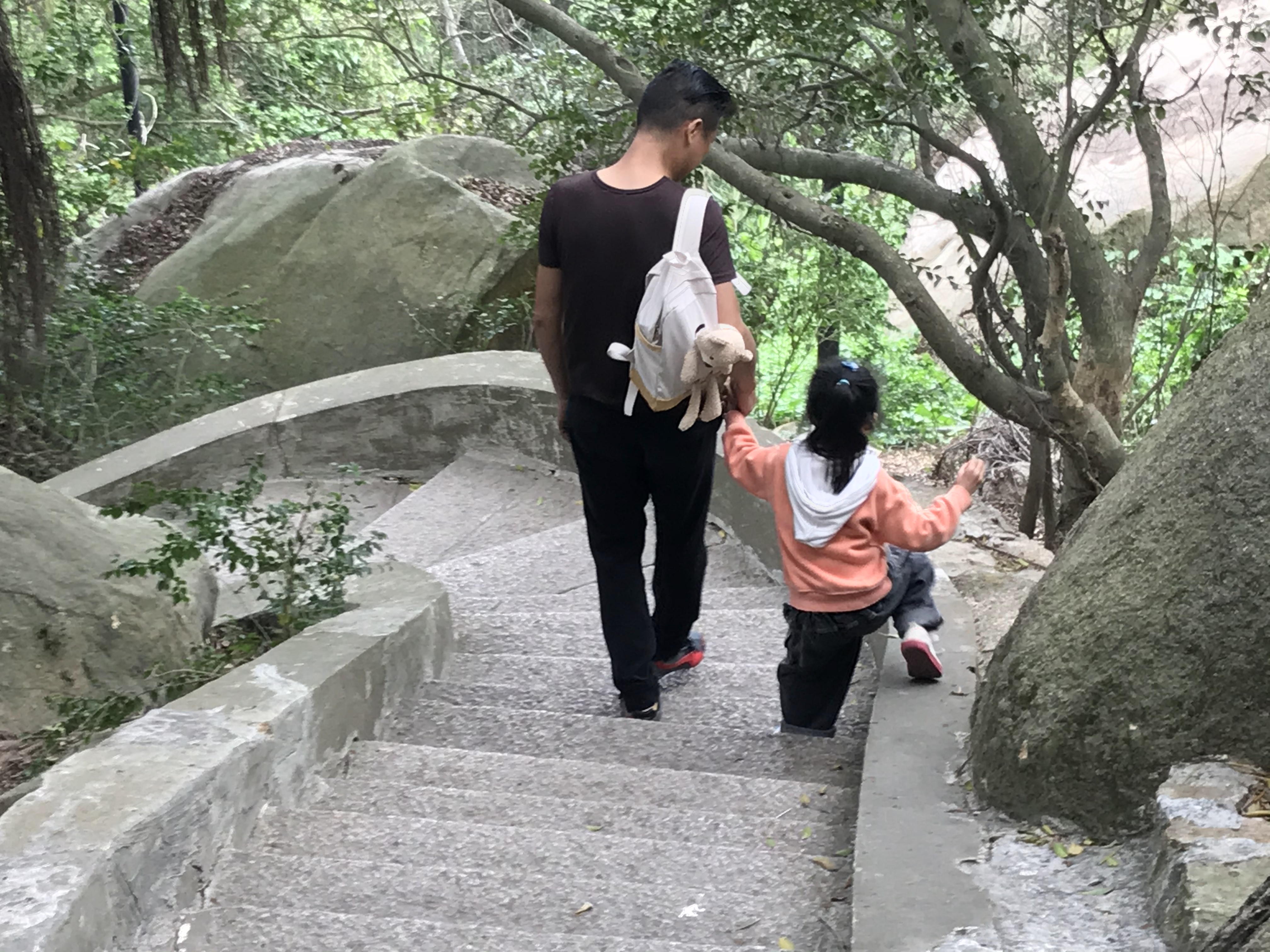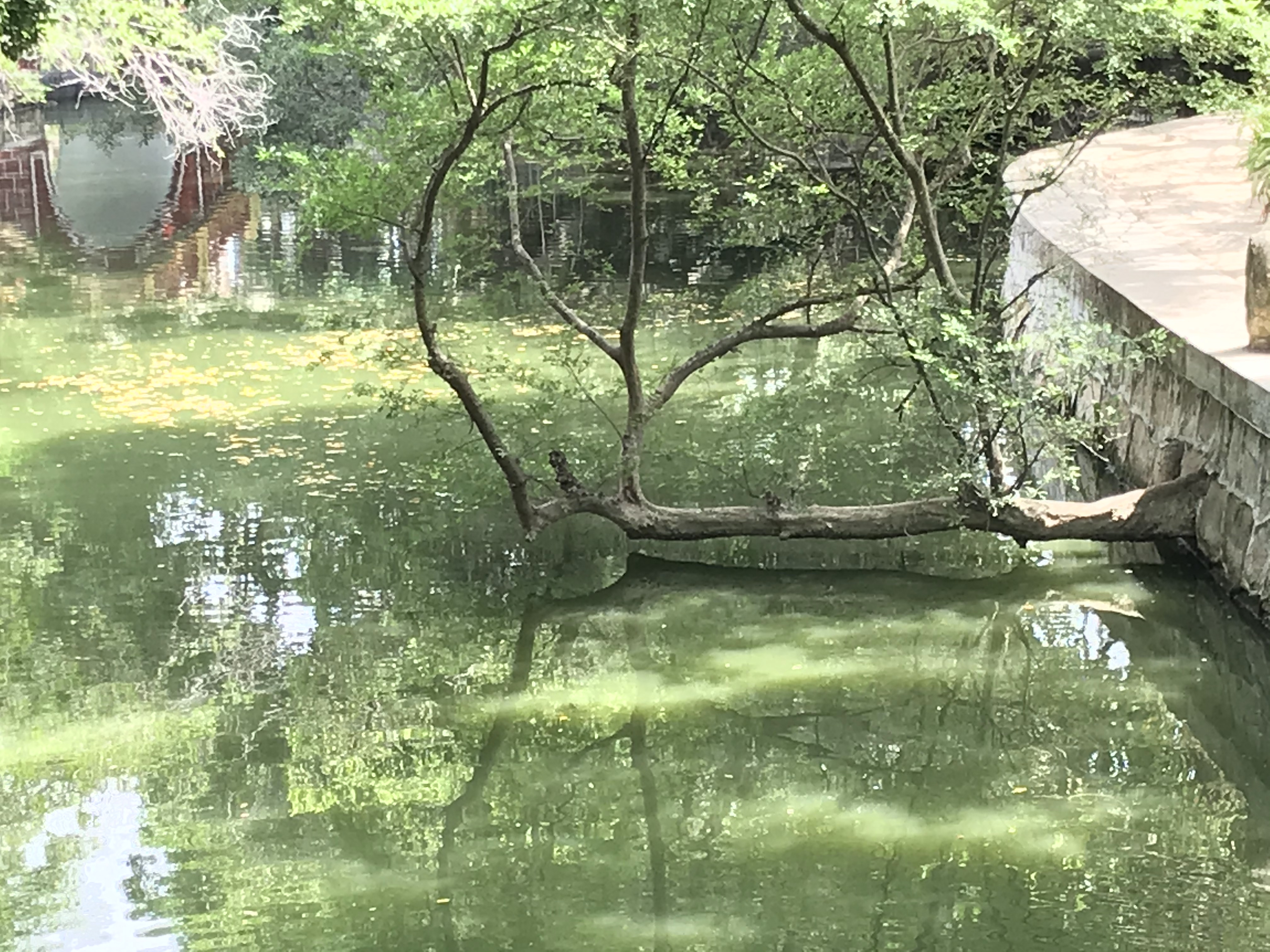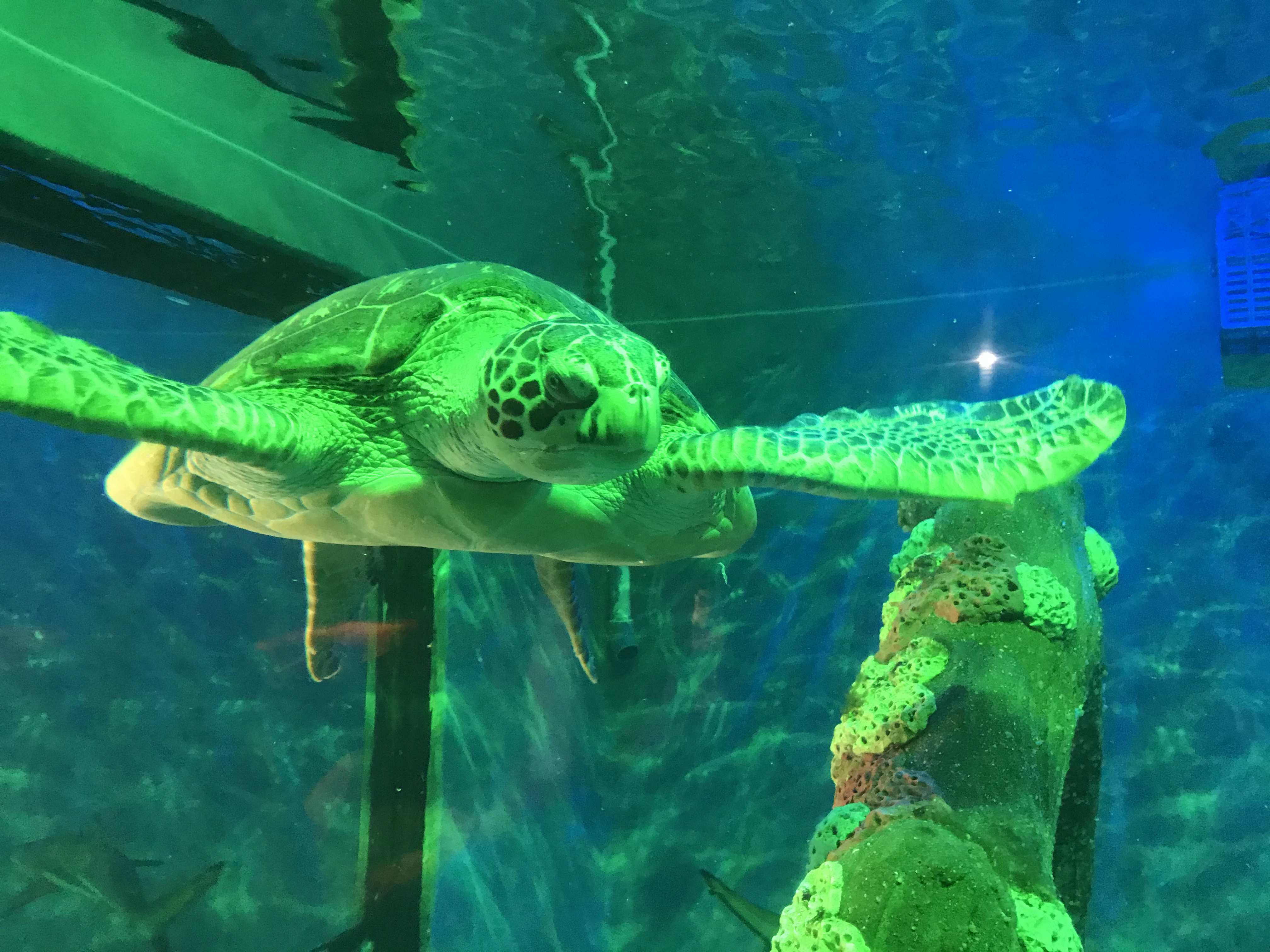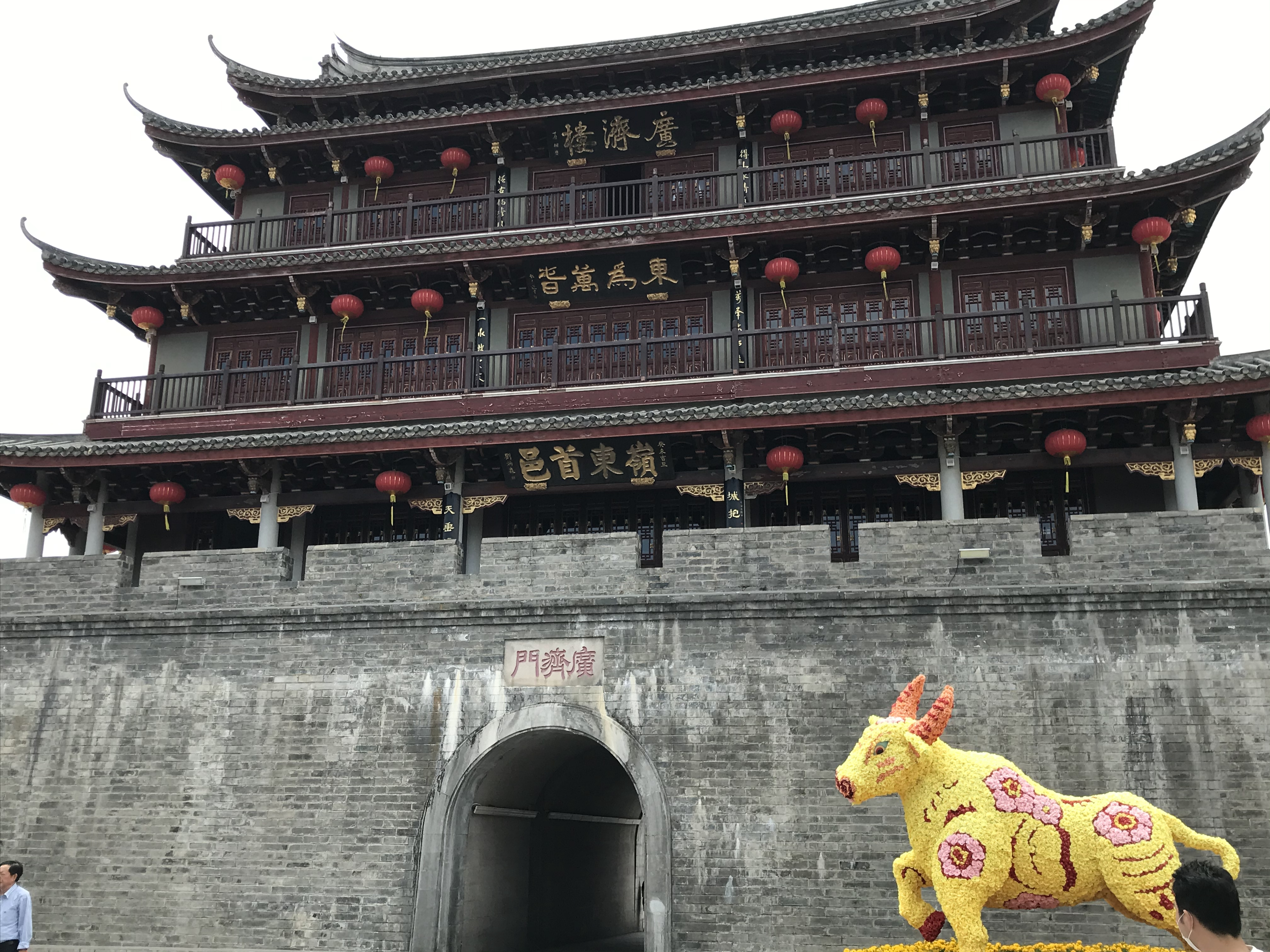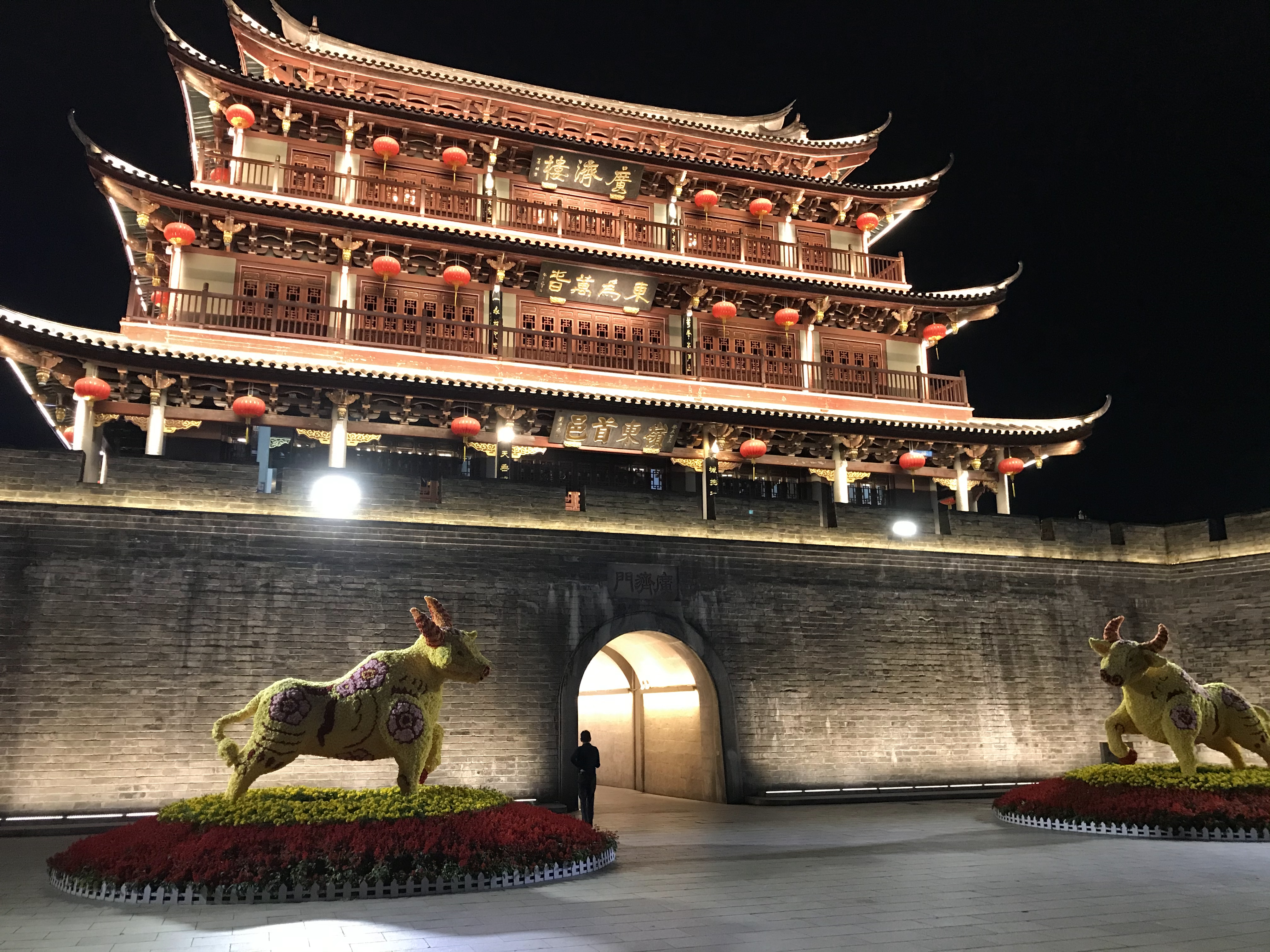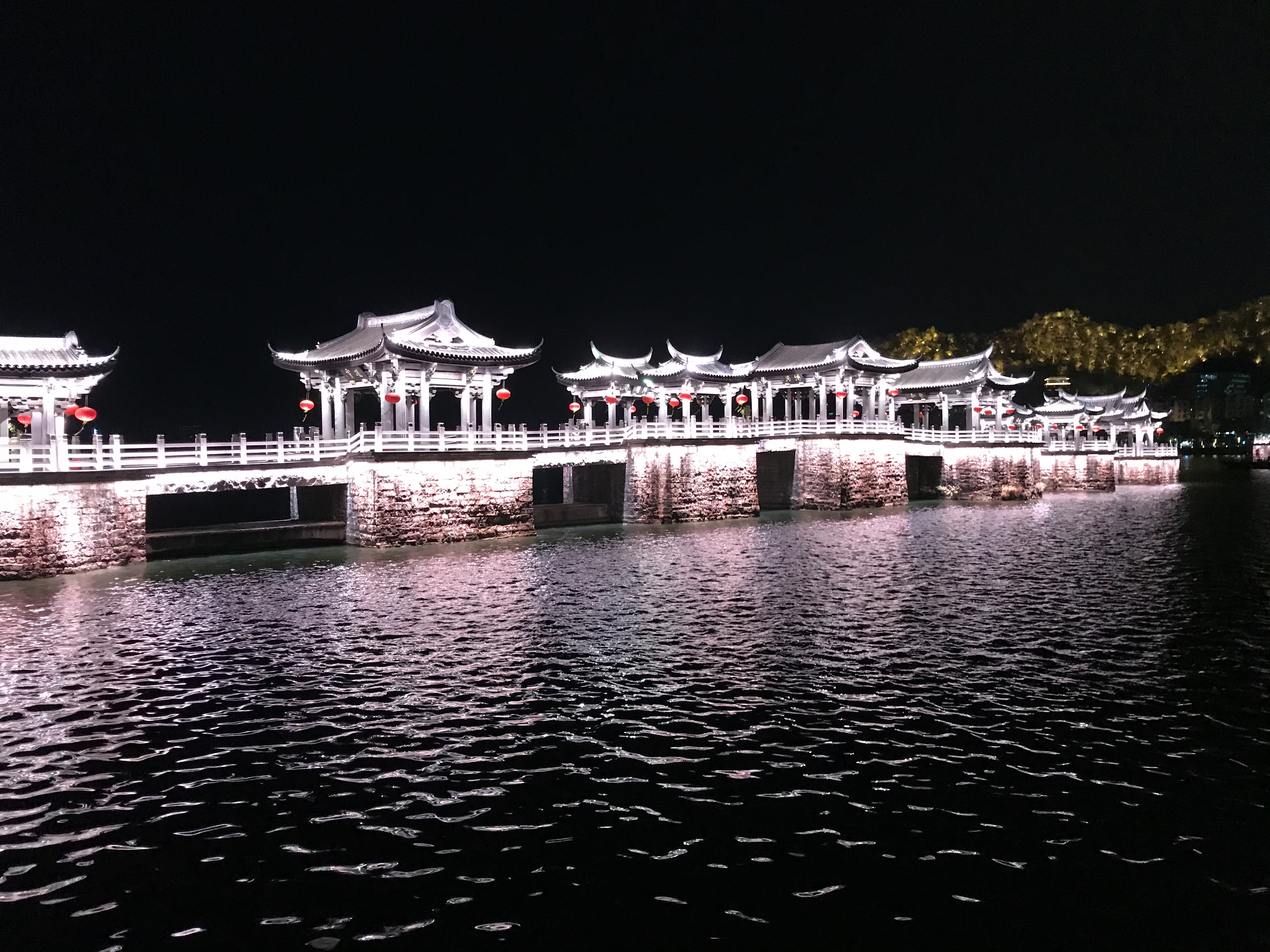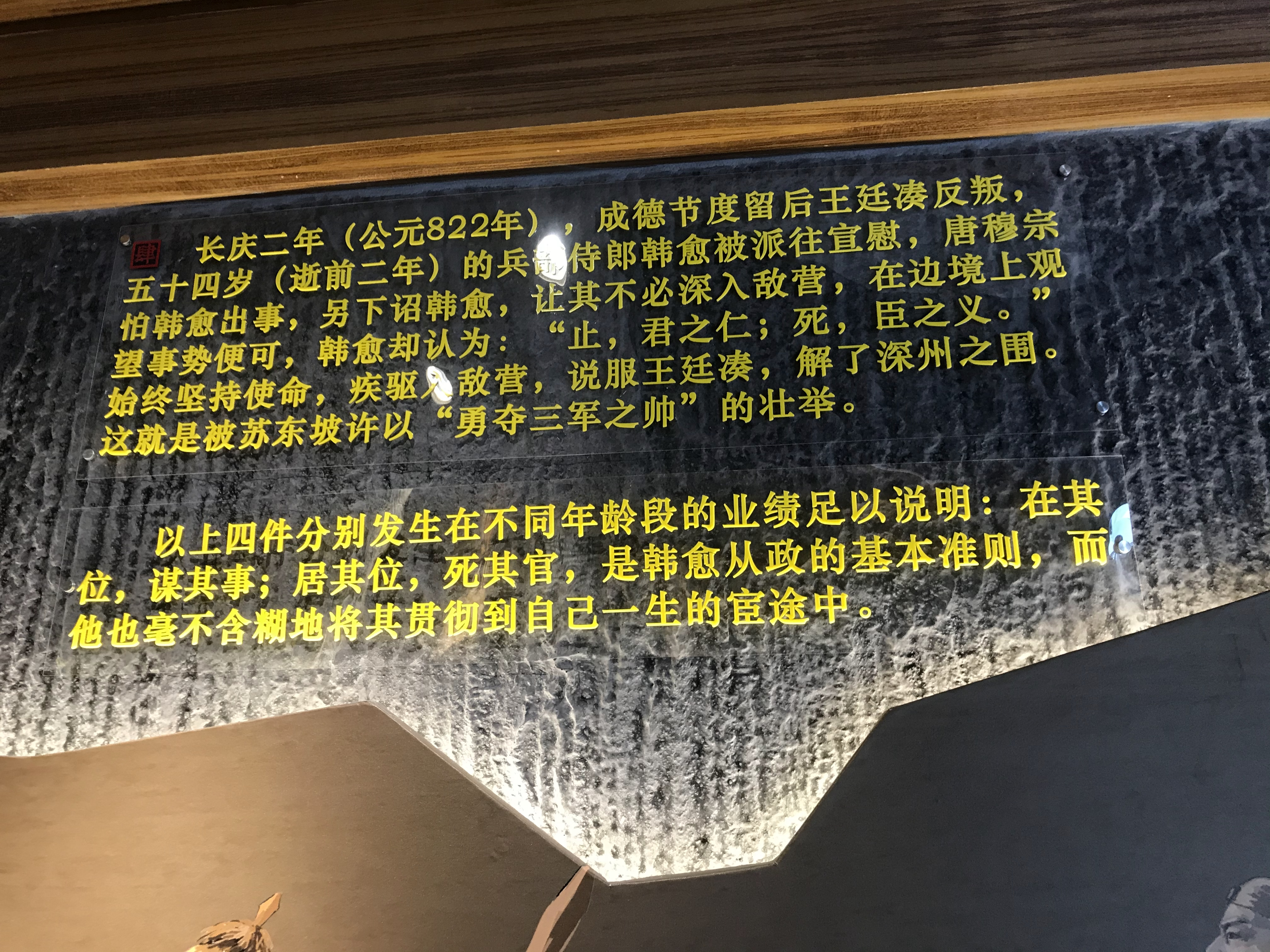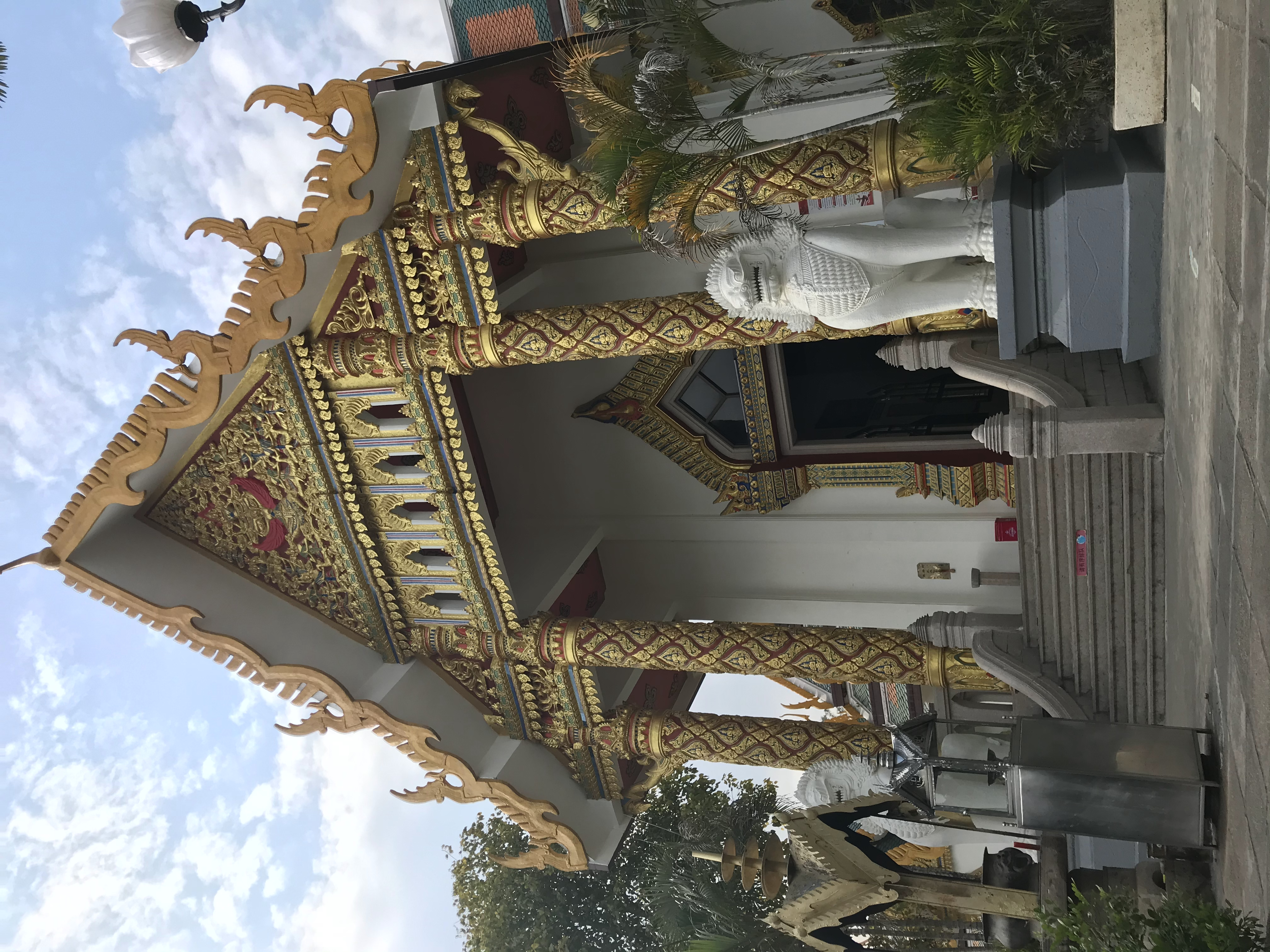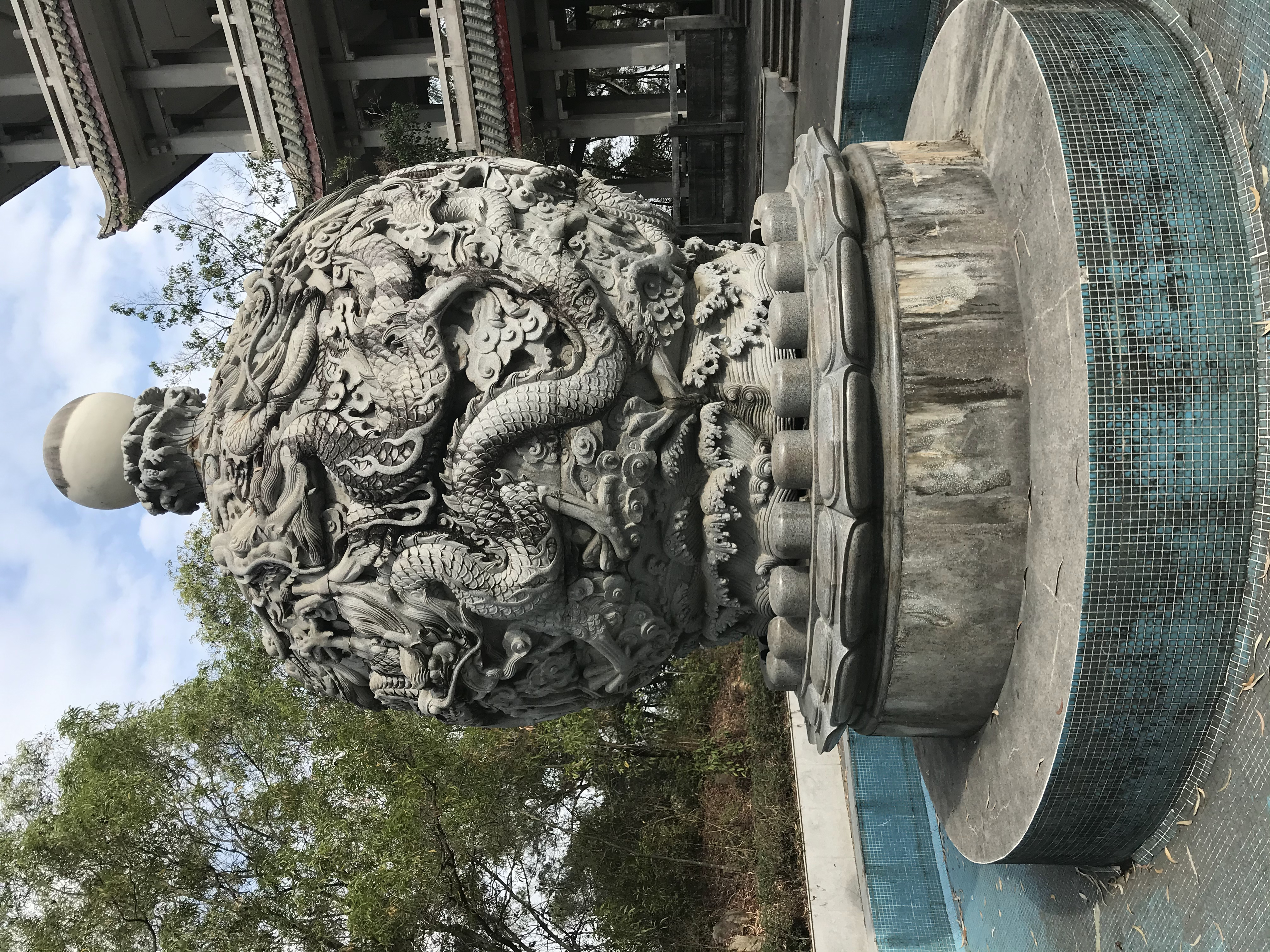# 旅行散记第一期——海的味道

Last updated on：8 months ago

# 二. 背景

1. ## 行程总览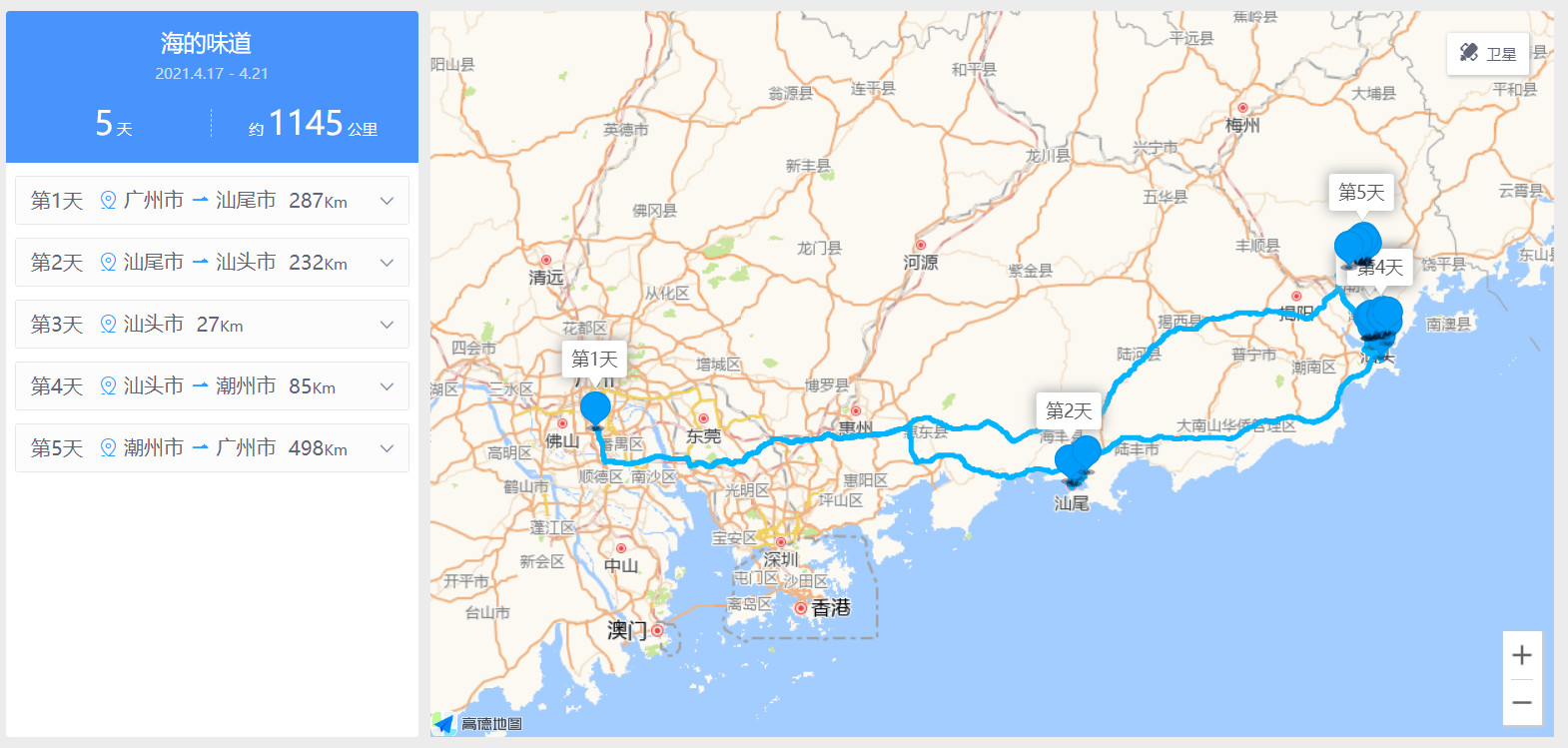# 三. 游途

graph LR
A[汕尾] -->B(汕尾站)
B --> C(汕尾市博物馆)
C --> D(东苑宾馆)
D -->|Day1| E(凤山祖庙旅游区)
E --> F(卫东渔业村)
F --> D
D -->|Day2| H(汕尾站)
H --> I[汕头]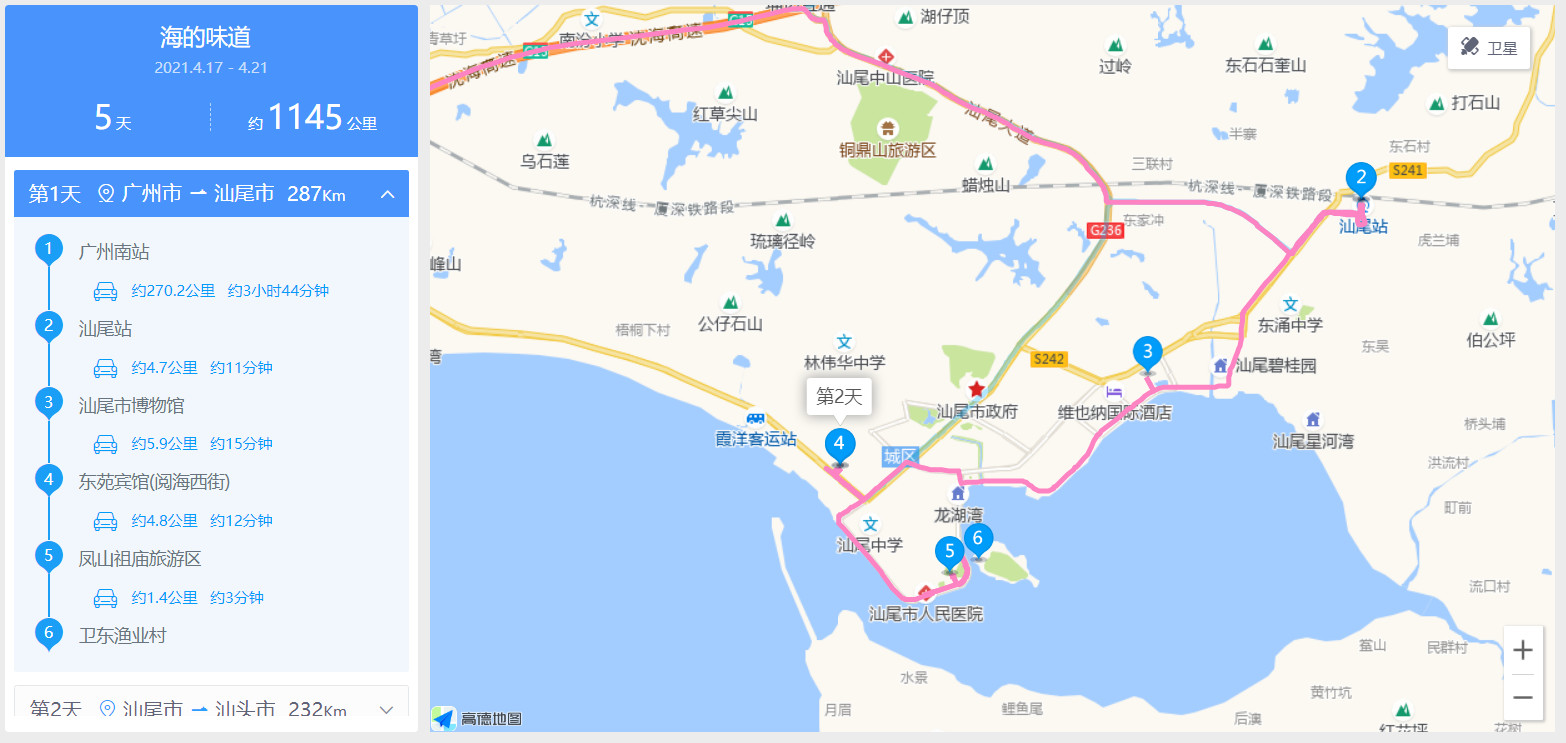graph LR
A[汕头] -->B(汕头站)
B --> C(石炮台公园)
C --> D(存心善堂)
D --> E(天后宫)
E --> F(老王牌炒粿糕)
F --> G(龙祥住宿)
graph LR
A[汕头续1] -->G(龙祥住宿)
G -->|Day2| H(礐石风景名胜区)
H --> I(达濠古城)
I --> G
G -->|Day3| J(汕头老城+小公园)
J --> L(十二中草莓冰红豆沙屋)
L --> N(中山公园+汕头两栖馆+动物园)
N --> O(7天酒店)
graph LR
A[汕头续2] -->G(7天酒店)
G -->|Day3| H(妈屿岛旅游区)
H --> J(珠江路美食街)
J --> K(小般口蚝烙)
K --> G
G -->|Day4| M(丹阳牛肉)
M --> N(汕头站)
N --> O[潮州]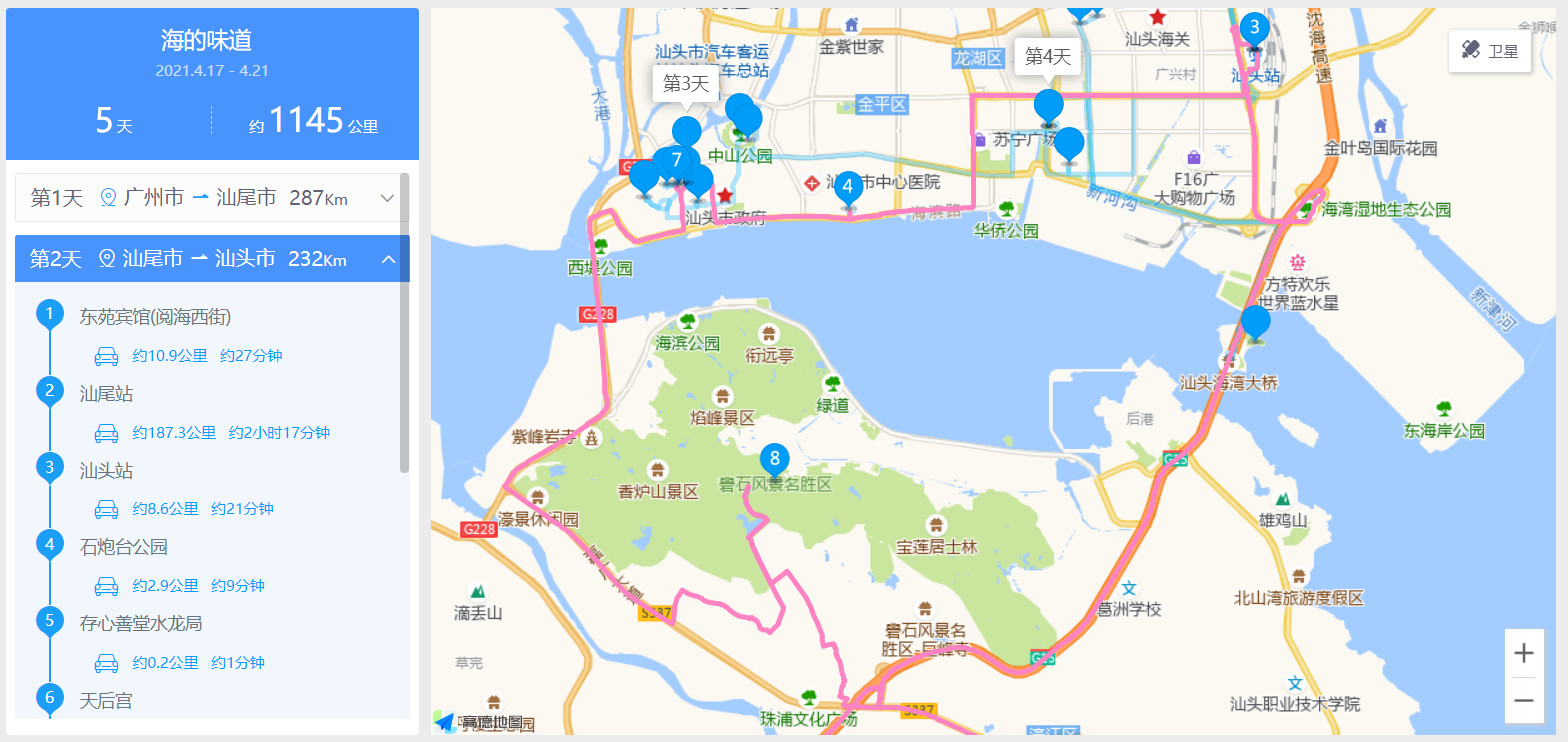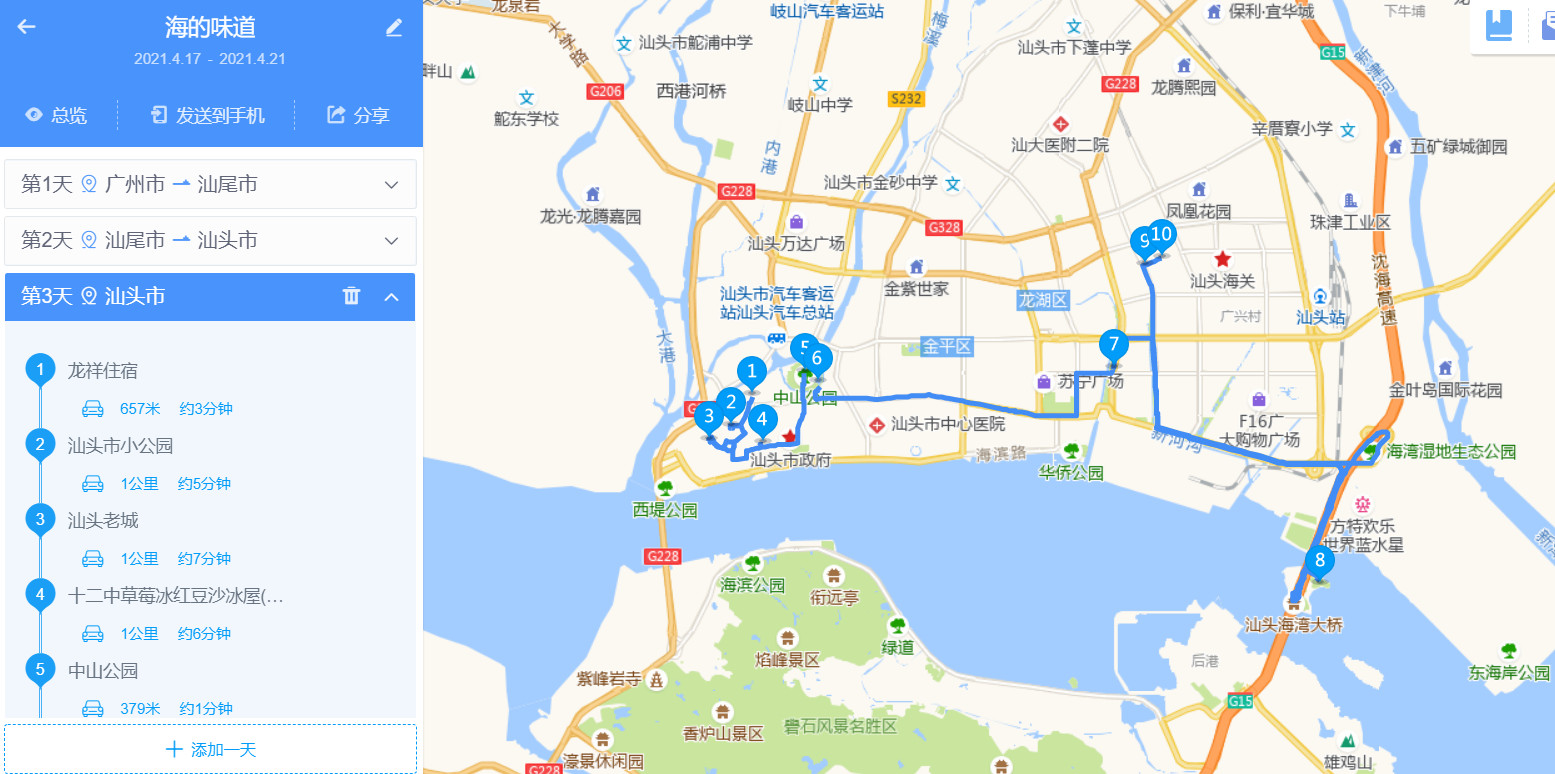graph LR
A[潮州] -->B(潮州站)
B --> C(人民广场+潮州博物馆)
C --> D(十八曲老尾鱼饺店)
D --> E(如家酒店)
graph LR
A[潮州续] -->E(如家酒店)
E -->|Day4| F(叩齿古寺)
F --> Z(老祖宗石磨坊)
Z --> H(开元寺)
H --> I(广济桥)
I --> J(韩愈纪念馆)
J --> K(开元寺泰佛殿)
K --> L(慧如公园)
L --> M(潮州西湖)
M --> E
E -->|Day5| N(许驸马府)
N --> O(北阁佛灯)
O --> P(潮州市湘桥区博物馆)
P --> Q(纽肉有约)
Q --> R(潮州站)
R --> S(汕头站)
S --> T(广州南站)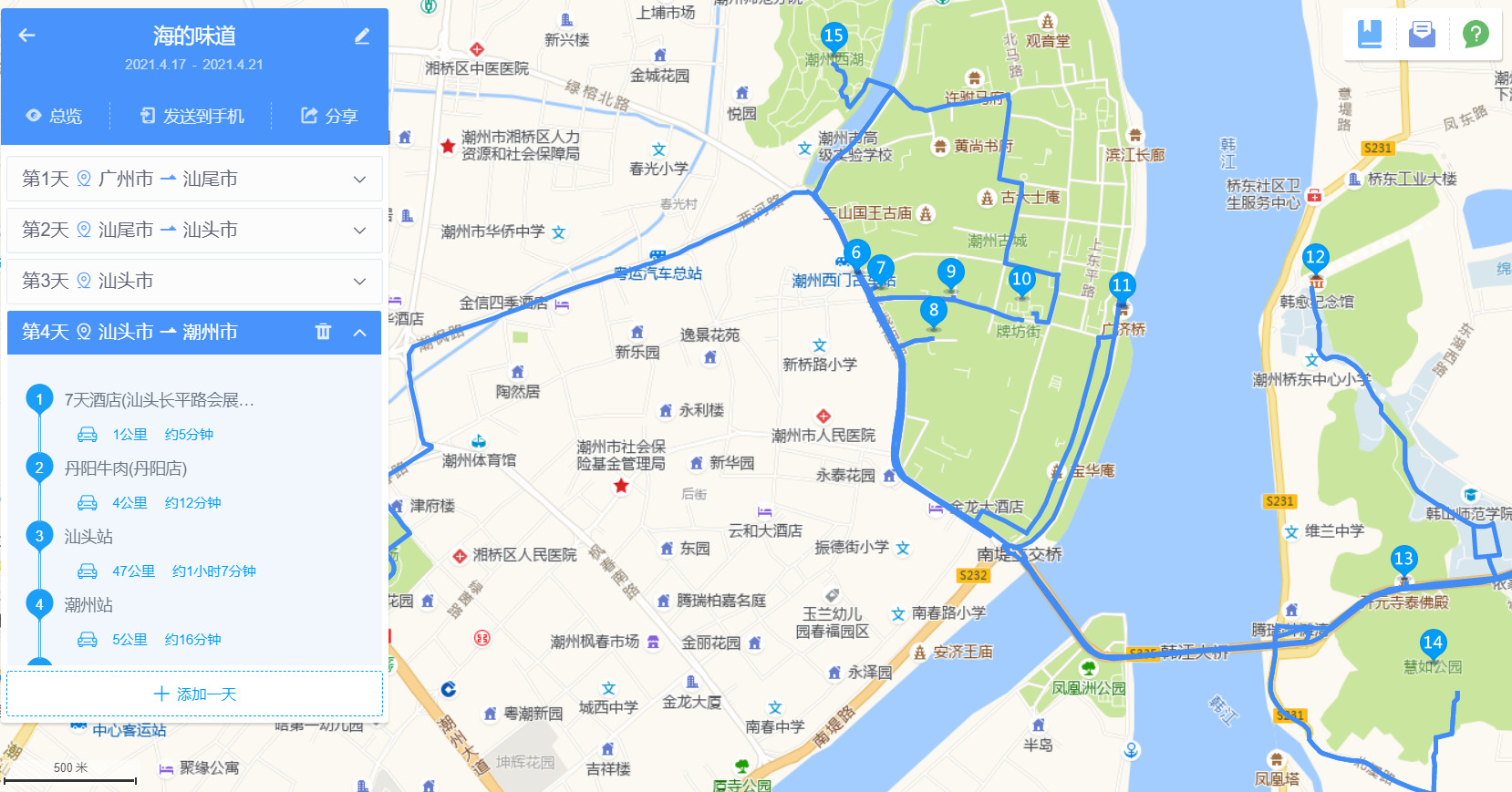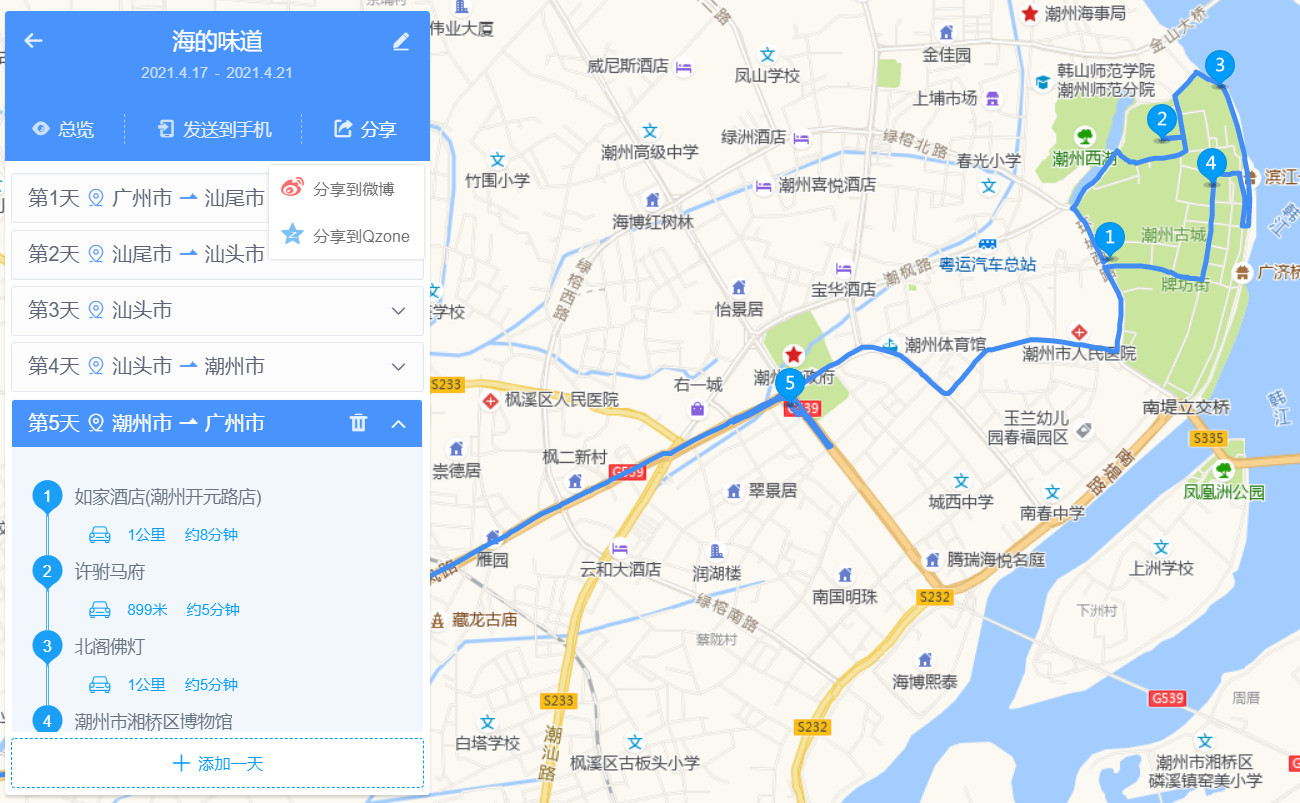# 四. 沿途风物照

1. ## 各种美食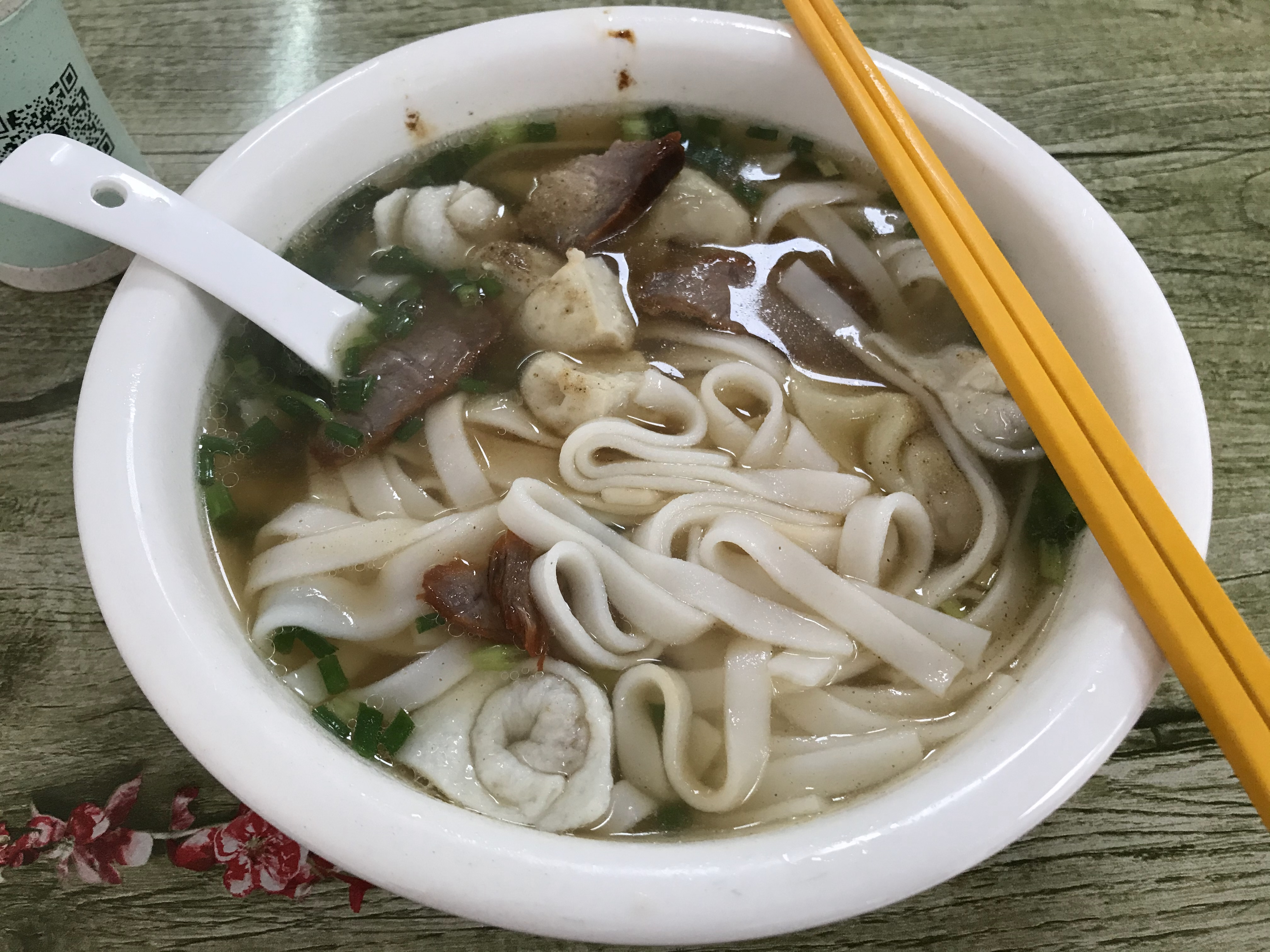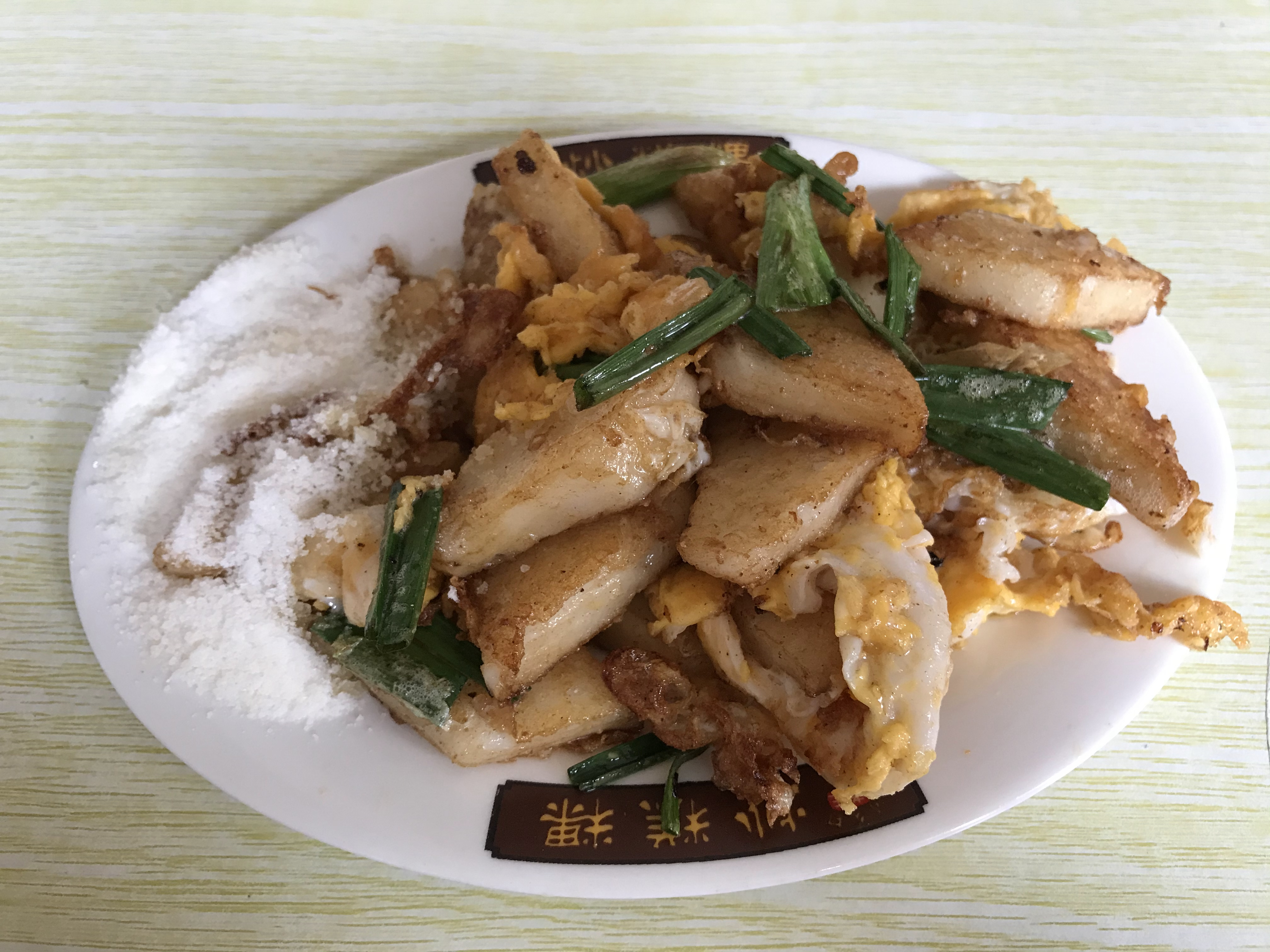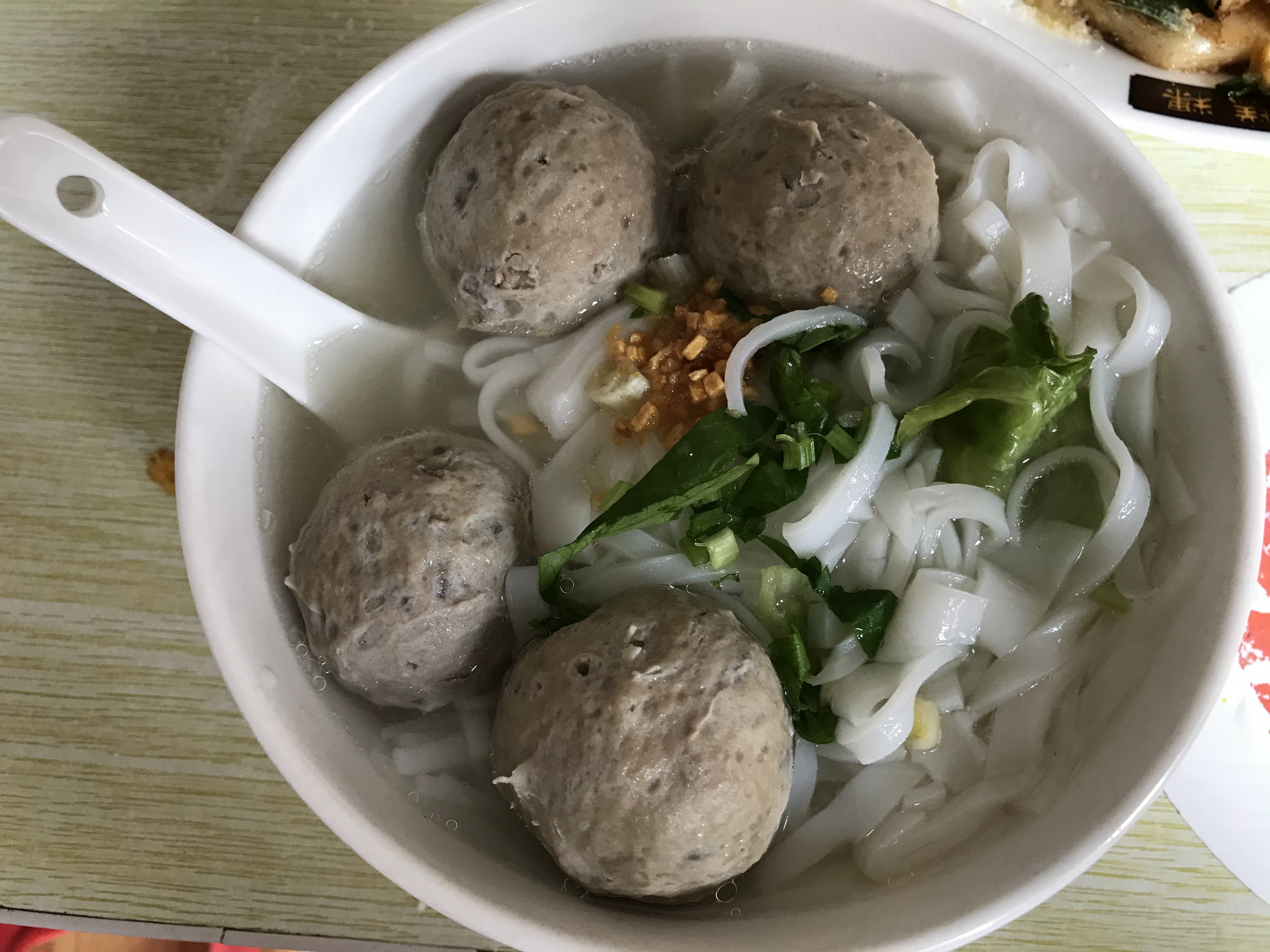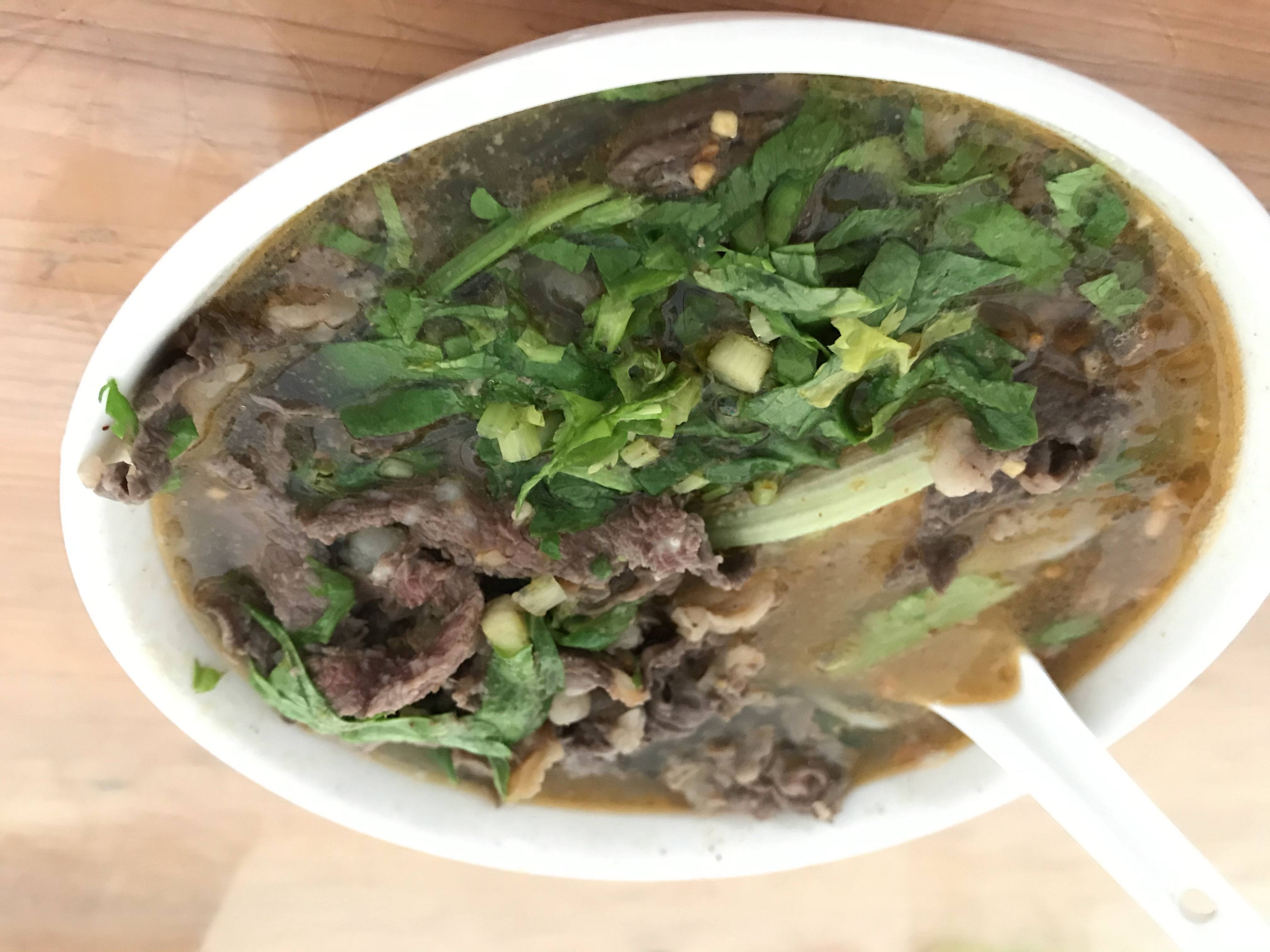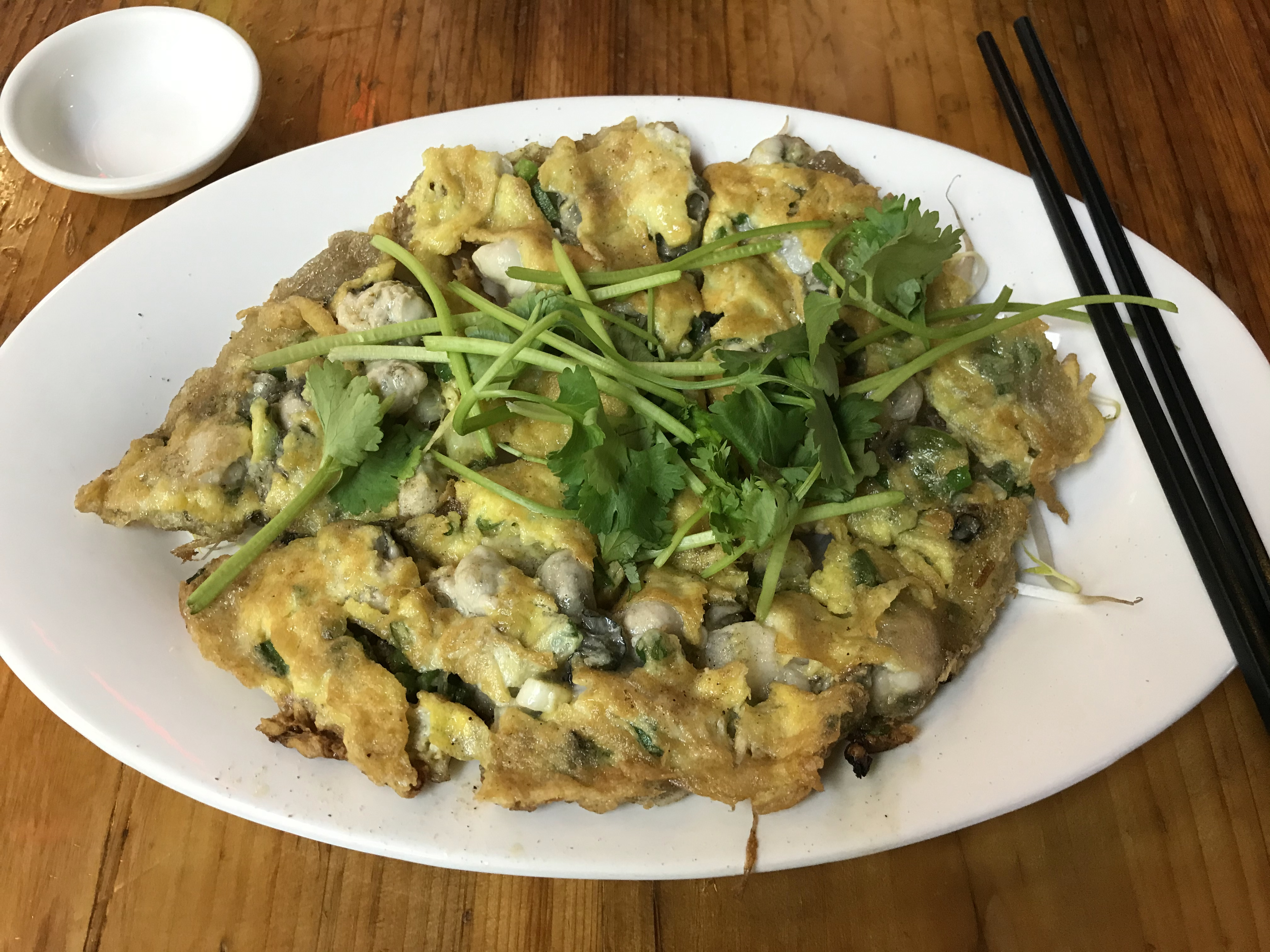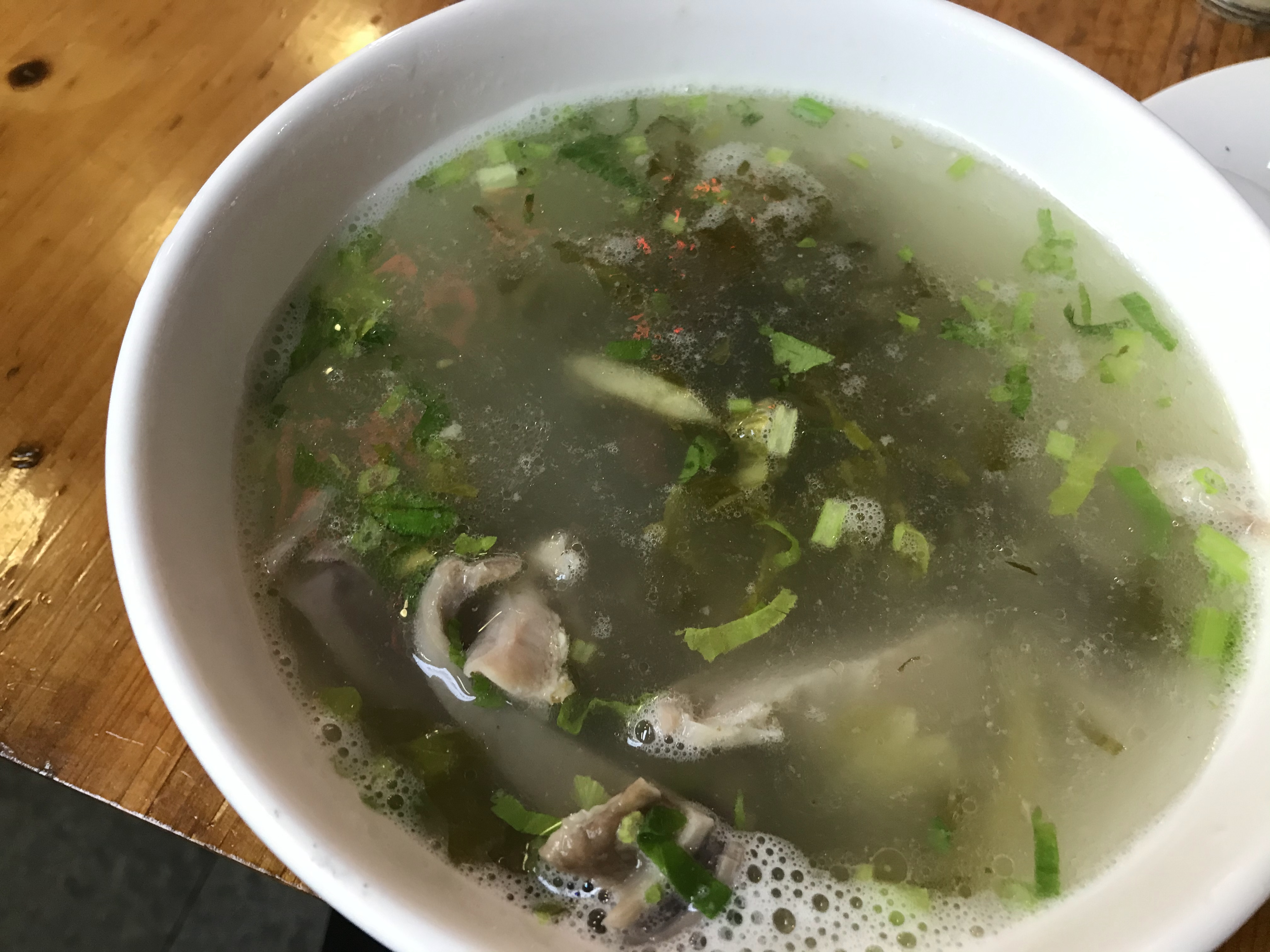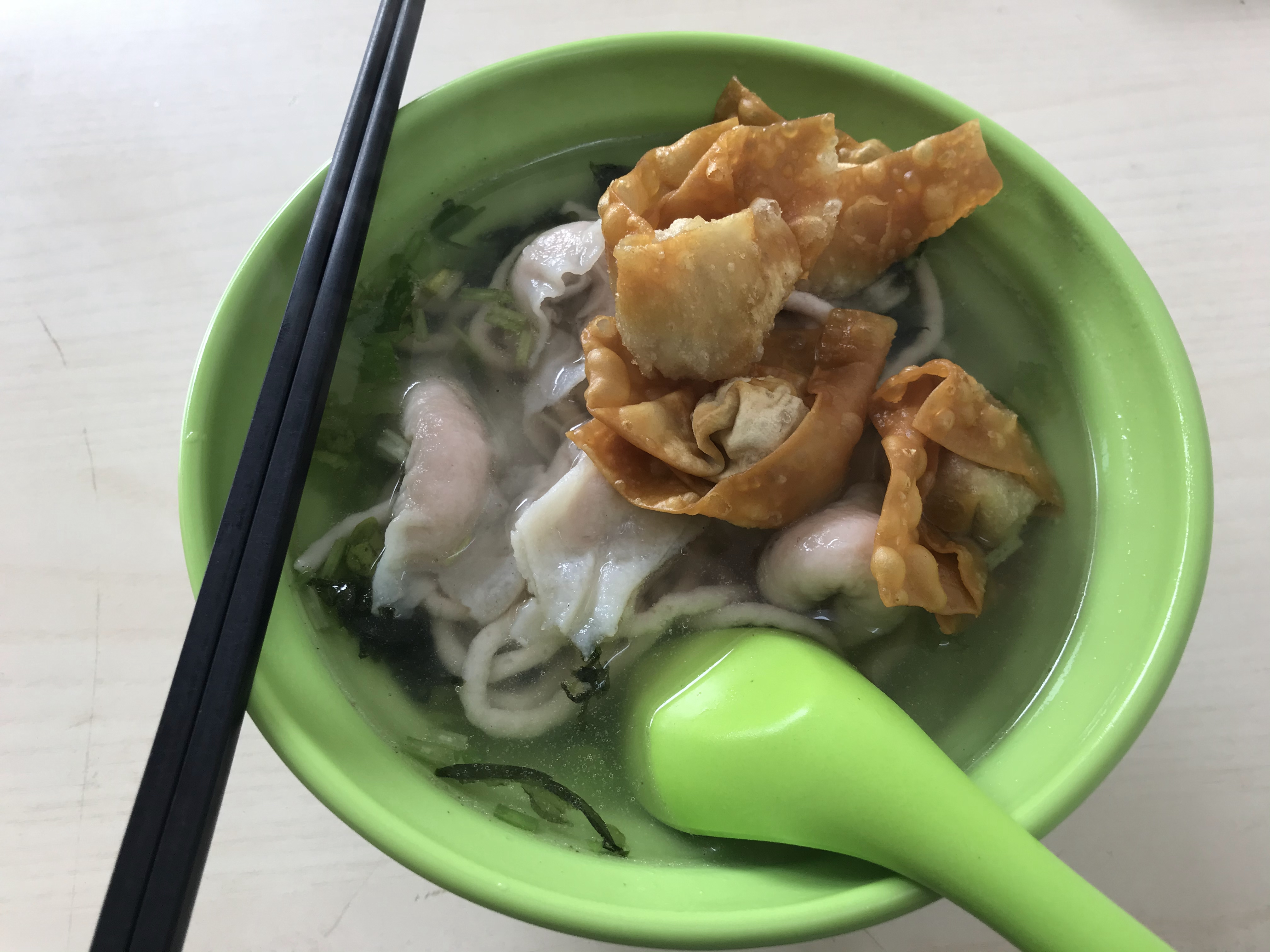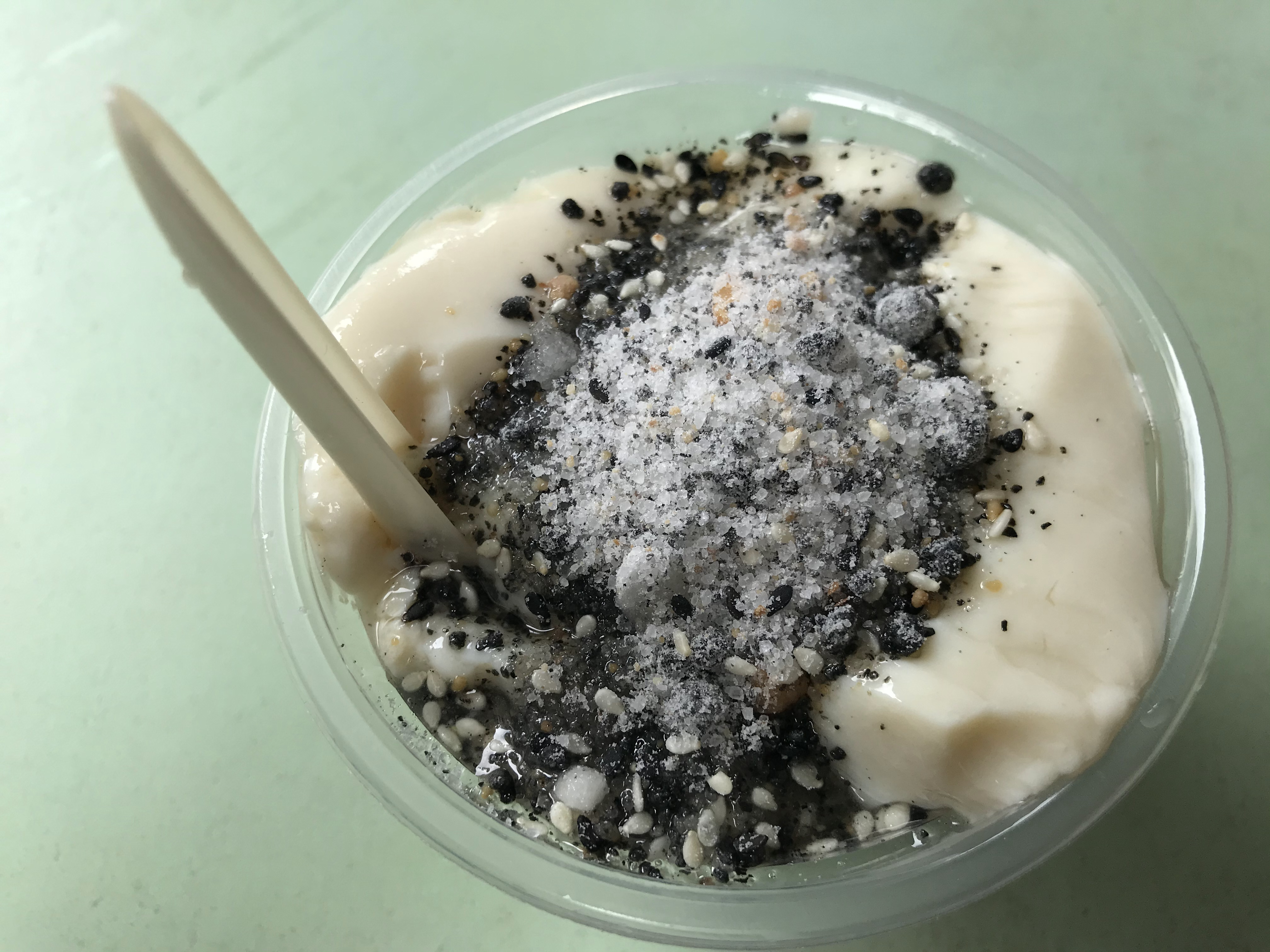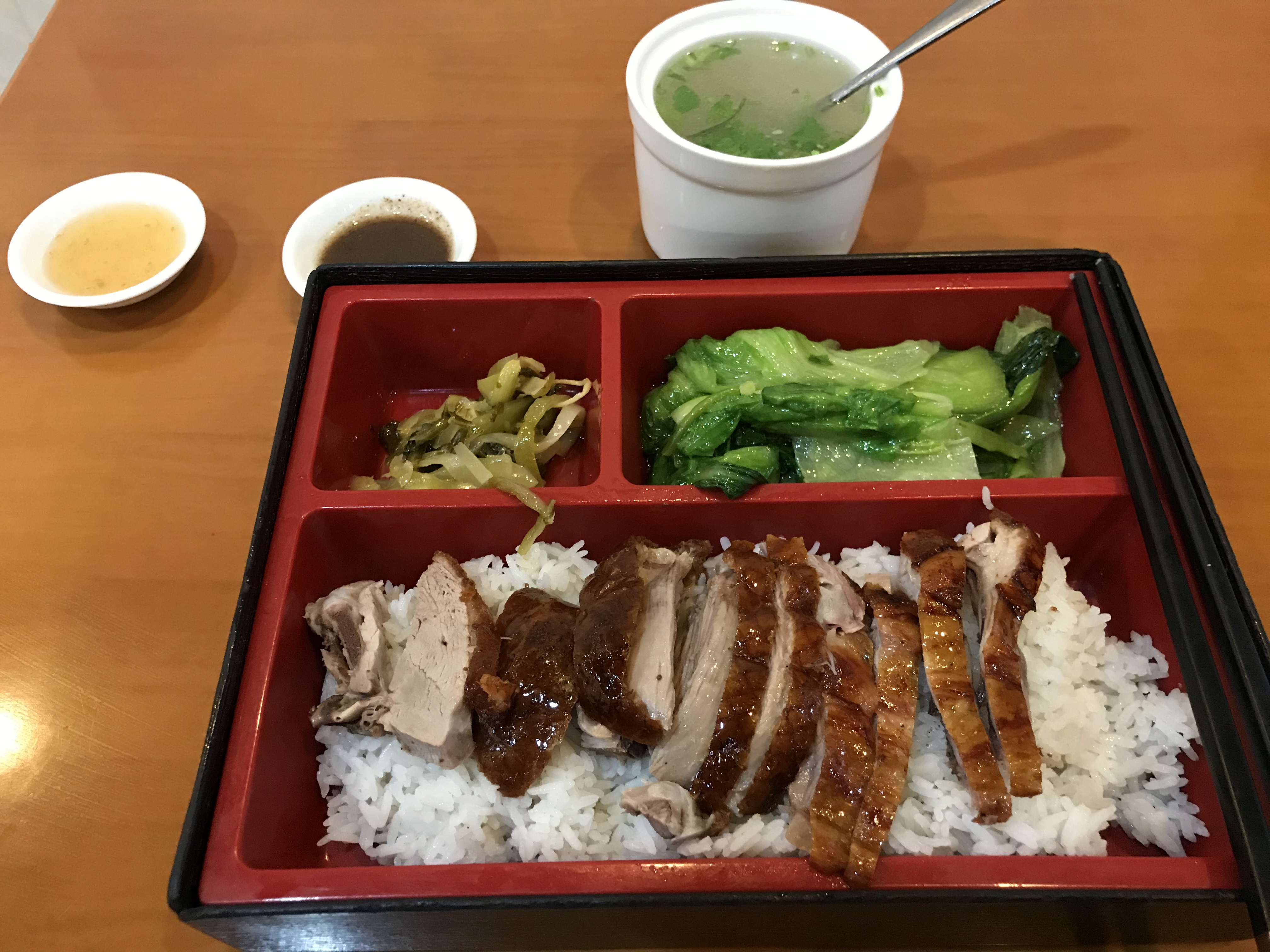1. ## 挑选景物